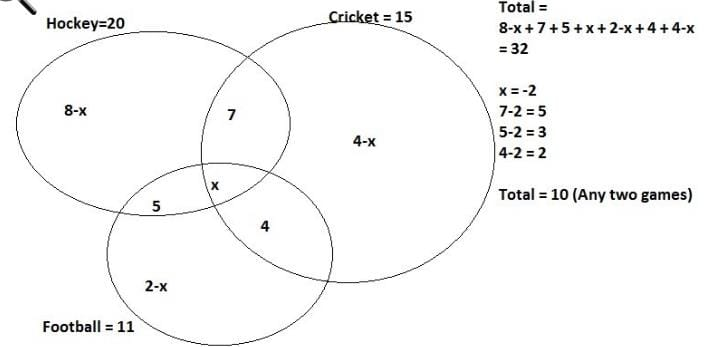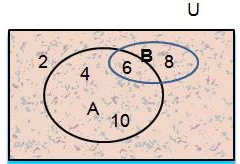JEE  >  Test: Counting Theorems

# Test: Counting Theorems

Test Description

## 17 Questions MCQ Test | Test: Counting Theorems

Test: Counting Theorems for JEE 2023 is part of JEE preparation. The Test: Counting Theorems questions and answers have been prepared according to the JEE exam syllabus.The Test: Counting Theorems MCQs are made for JEE 2023 Exam. Find important definitions, questions, notes, meanings, examples, exercises, MCQs and online tests for Test: Counting Theorems below.
Solutions of Test: Counting Theorems questions in English are available as part of our course for JEE & Test: Counting Theorems solutions in Hindi for JEE course. Download more important topics, notes, lectures and mock test series for JEE Exam by signing up for free. Attempt Test: Counting Theorems | 17 questions in 15 minutes | Mock test for JEE preparation | Free important questions MCQ to study for JEE Exam | Download free PDF with solutions
 1 Crore+ students have signed up on EduRev. Have you?
Test: Counting Theorems - Question 1

### If n (P) = 5, n(Q) = 12 and n(P U Q) = 14 then n(P ∩ Q) =

Detailed Solution for Test: Counting Theorems - Question 1

n (P) = 5, n(Q) = 12 and n(PUQ) = 14
n(PUQ) = n(P) + n(Q) - n(P∩Q)
14 = 5 + 12 + - n(P∩Q)
n(P∩Q) = 3

Test: Counting Theorems - Question 2

### In a class of 50 students, 20 play Hockey, 15 play Cricket and 11 play Football. 7 play both Hockey and Cricket, 4 play Cricket and Football and 5 play Hockey and football. If 18 students do not play any of these given sports, how many students play exactly two of these sports?

Detailed Solution for Test: Counting Theorems - Question 2Test: Counting Theorems - Question 3

### Let A and B be two finite disjoint sets such that n(A ∪ B) = 475 and n(A) = 435, then n(B) is

Detailed Solution for Test: Counting Theorems - Question 3

As a and b are disjoint sets, they have no elements in common. Thus,
n(a∪b) = n(a) + n(b)
→n(b) = n(a∪b) − n(a)
= 475 − 435
= 40

Test: Counting Theorems - Question 4

If n(A) + n(B) + n(C) = n(A U B U C) then what are the sets A, B, C are called?

Detailed Solution for Test: Counting Theorems - Question 4

A and B are disjoint set so their is no common element between A and B.
n(AuBuC) = n(A)+n(B)+ n(C)-n(AnB)-n(Anc)-n(BnC)+n(AnBnC)
As we know n(AuB)=n(A)+ n(B)-n(AnB)
Here when we add A and B we are adding (AnB) two times , as it is present in both A and B. So we subtract (AnB) one time from A and B.
Which means we need to take the area of A and B set but no area should repeat.
When we take three sets A,B and C
We take area of A+ Area of B + area of C ————eq
But they are intersecting so the area we are taking is greater than actual area. We have again added (AnB), (AnC) and (BnC) twice. Therefore we subtract the intersecting area from eq one time as done above
A+B+C-(AnB)-(AnC)-(BnC)

The intersection of the sets a and b is the set of all the elements which belong to both A and B. It is denoted by A ∩ B (“A intersection B”).
• If A and B do not have any element in common then A ∩ B= a null set = Ø.

Test: Counting Theorems - Question 5

If in class XI of a certain school, 24 students got distinction in mathematics, 19 students got distinction in physics and 15 students got distinction in both the subjects, then the number of students in class XI is:

Detailed Solution for Test: Counting Theorems - Question 5

r :- c
Explanation:- Students got distinction in mathematics = 24
Student got distinction in physics = 19
Students got distinction in both= 15
Total number of students = (24+19)-15
= 28

Test: Counting Theorems - Question 6

There is a group of 80 persons who can drive scooter or car or both. Out of these, 35 can drive scooter and 60 can drive car. Find how many can drive both scooter and car? How many can drive scooter only? How many can drive car only?(Respectively)

Detailed Solution for Test: Counting Theorems - Question 6

Let S = {Persons who drive scooter}
C = {Persons who drive car}
Given, n(S ∪ C) = 80, n(S) = 35, n(C) = 60
Therefore, n(S ∪ C) = n(S) + n(C) - n(S ∩ C)
80 = 35 + 60 - n(S ∩ C)
80 = 95 - n(S ∩ C)
Therefore, n(S ∩ C) = 95 – 80 = 15
Therefore, 15 persons drive both
scooter and car.
Therefore, the number of persons who
drive a scooter only = n(S) - n(S ∩ C)
= 35 – 15
= 20
Also, the number of persons who drive car only = n(C) - n(S ∩ C)
= 60 - 15
= 45

Test: Counting Theorems - Question 7

If P and Q are two sets such that n(P) = 120, n(Q) = 50 and n(P ∪ Q) = 140 then, n(P ∩ Q) is:

Test: Counting Theorems - Question 8

If two sets A and B are having 99 elements in common, then the number of elements common to each of the sets× B and× A are

Detailed Solution for Test: Counting Theorems - Question 8

n((× B) ∩ (× A)) = n((∩ B) × (∩ A)) = n(∩ B).n(∩ A)
n(∩ B).n(∩ B) = (99).(99) = 992.

Test: Counting Theorems - Question 9

If U = 100, n(A) = 30, n(B) = 40, n(A ∩ B) = 10, then n( A' ∩ B' ) =

Detailed Solution for Test: Counting Theorems - Question 9

By demorgan's law
n( A' ∩ B' )=n(A ∪ B)' = n(U) - n(A ∪ B)
=100 - [ n(A) + n(B) - n(A ∩ B) ]
=100 - 60 = 40

Test: Counting Theorems - Question 10

The number of non-empty subsets of the set {1, 2, 3, 4} is

Detailed Solution for Test: Counting Theorems - Question 10

The number of non- empty subsets =
2n − 1 = 24 − 1 = 16 − 1 = 15

Test: Counting Theorems - Question 11

A group of 120 students, 90 take mathematics and 72 take economics. If 10 students take neither of the two, how many students take both:

Detailed Solution for Test: Counting Theorems - Question 11

P(U) = 120
Mathematics = P(A) = 90
Economics = P(B) = 72
P(A ∪ B)' = 10
P(A ∪ B) = P(U) - P(A ∪ B)'
= 120 - 10 = 110
P(A ∪ B) = P(A) + P(B) - P(A ∪ B)
110 = 90 + 72 -P(A ∩ B)
P( A ∩ B) = 162 - 110 = 52

Test: Counting Theorems - Question 12

In a class of 50 students, 35 students play cricket and 30 students play football; identify the students playing both the games.

Test: Counting Theorems - Question 13

In a class of 40 students 14 take physics and 29 take chemistry. If 5 students take both, how many students take neither of the subjects?

Detailed Solution for Test: Counting Theorems - Question 13

Total students who took any of the subjects = 40 - x
Let x be the no. of students who took neither of the subjects
Then, 40 - x = 14 + 29 - 5
⇒ x = 2

Test: Counting Theorems - Question 14

If A = {1, 4, 9, 16} then n (A) = _____

Detailed Solution for Test: Counting Theorems - Question 14

N(A) indicates the number of elements in set A, there are 4 elements.

Test: Counting Theorems - Question 15

Find n (A – B) from the figureDetailed Solution for Test: Counting Theorems - Question 15

A={4,6,10} B={6,8}
A-B={4,10}
= n(A-B) = 2 Elements

Test: Counting Theorems - Question 16

A,B,C are three sets such that n(A) = 25, n(B) = 20, n(c) = 27, n(A ∩ B) = 5, n(B ∩ C ) = 7 and A ∩ C = ∅ then n(A ∪ B ∪ C) is equal to

Detailed Solution for Test: Counting Theorems - Question 16

Here A ∩ C = Ø ⇒ A ∩ B ∩ C = Ø
n (A ∪ B ∪ C) = n(A) + n(B) + n(C) - n(A ∩ B) - n(B ∩ C) - n(C ∩ A) + n(A ∩ B ∩ C)
= 25 + 20 + 27 - 5 - 7 - 0 - 0
= 60

Test: Counting Theorems - Question 17

In a factory 70% of the workers like oranges and 64% like apples. If x% like both oranges and apples, then

Detailed Solution for Test: Counting Theorems - Question 17

let the total number of workers be 100. A = who likes oranges , B = who likes apples
So n(A ) = 70 and n(B) = 64, n(A ∩ B) = x
Also n(A U B) ≤ 100
n(A) + n(B) -n(A ∩ B) ≤ 100
70 + 64 - x ≤ 100
x ≥ 34
and n(A ∩ B) ≤ n(B) ⇒ x ≤ 64
then 34 ≤ x ≤ 64

Information about Test: Counting Theorems Page
In this test you can find the Exam questions for Test: Counting Theorems solved & explained in the simplest way possible. Besides giving Questions and answers for Test: Counting Theorems , EduRev gives you an ample number of Online tests for practice(Scan QR code)